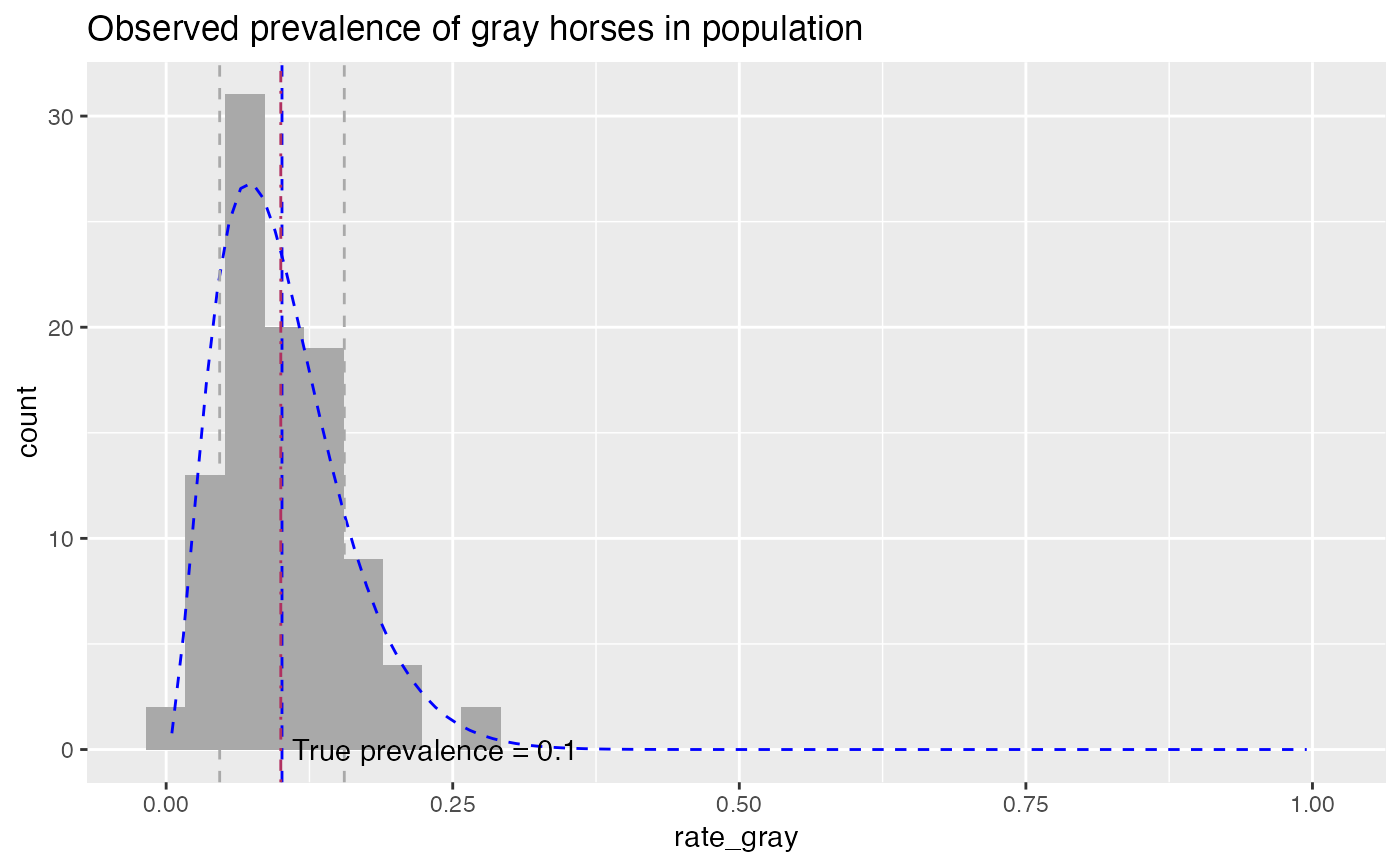Compares empirical rate data to a beta distribution with the same mean and standard deviation.

PlotDistHistBeta(
frm,
xvar,
title,
...,
bins = 30,
hist_color = "darkgray",
beta_color = "blue",
mean_color = "blue",
sd_color = "darkgray"
)

## Arguments

frm data frame to get values from name of the independent (input or model) column in frame title to place on plot force later arguments to bind by name passed to geom_histogram(). Default: 30 color of empirical histogram color of matching theoretical beta color of mean line color of 1-standard devation lines (can be NULL)

ggplot2 plot

## Details

Plots the histogram of the empirical distribution and the density of the matching beta distribution. Also plots the mean and plus/minus one standard deviation.

The number of bins for the histogram defaults to 30. The binwidth can also be passed in instead of the number of bins.

## Examples


set.seed(52523)
N = 100
pgray = 0.1  # rate of gray horses in the population
herd_size = round(runif(N, min=25, 50))
ngray = rbinom(N, herd_size, pgray)
hdata = data.frame(n_gray=ngray, herd_size=herd_size)

# observed rate of gray horses in each herd
hdata\$rate_gray = with(hdata, n_gray/herd_size)

title = "Observed prevalence of gray horses in population"

PlotDistHistBeta(hdata, "rate_gray", title) +
ggplot2::geom_vline(xintercept = pgray, linetype=4, color="maroon") +
ggplot2::annotate("text", x=pgray+0.01, y=0.01, hjust="left",
label = paste("True prevalence =", pgray))# no sd lines
PlotDistHistBeta(hdata, "rate_gray", title,
sd_color=NULL)